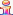﻿ <<*Set*>> Command

# <<*Set*>> Command

Sometimes you need to set a value. To do so, use the <<*Set*>> command.

When <<*Set*>>-ing values, you are assigning a value associated with a !groupname! without using other Pathagoras equations. (<<*Options*, *Optional*, *Repeat*, *AskOptions*, *AskOptional* or *AskRepeat* commands all 'set' values, but there may be circumstances where you want to <<*Set*>> a value directly.)

The formula is "<<*Set*!groupname!=" followed by a value. The value can either be:

"True", or "Yes" (for a 'true' type value)

"False, "No" (for a 'false type value)

A number > 0 when setting a 'repeat value' for the !groupname!

A positional value when you are setting a selection for a multiple choice item. (See special requirements in below examples.)

A single word value.

NOTE: The quotes around the value to which the group name is  being <<*Set*>> are mandatory.

Consider this:

When answered, the value assigned to !Married! is either "True" or "False" (or "Yes" or No").

You can directly set this value in a document where, avoiding the 'Is our client married?' question altogether (if the situation so calls) in the following manner:

<<*Set*!Married!="True">>
<<*Set*!Married!="Yes">> This is the functional equivalent of the above line.

You can also use a value set by an <<*AskOptions*>> or <<*AskOptions(radio)*>> command to set another value. Here's the setup, based on two MultiChoice lists. The alias of the first MultiChoice list is *States*. It contains 50 elements (the 50 United States of America). The second alias is called *SecurityType*. It, too, contains 50 elements. The values of these elements are the type of security instrument ('mortgage' or 'deed of trust') associated with each of the 50 States. The goal is to automate the selection of "Deed of Trust' or 'Mortgage', depending upon the state in which the transaction is occurring.

The AskOptions below (first line) sets the value of the groupname !st! to the value the user selects from the list of the 50 States when presented in the Interview. Once the State is selected, Pathagoras can then the value of the groupname called !Security! to the parallel position as the selected state. (So, if Indiana, the 14th position in the list of *States*, is selected, the value of !st! would be '14'. Then Pathagoras processes the !security! groupname by looking at the !st! position in the *SecType*alias list. "Mortgage" occupies that 14th position, and therefore Mortgage is assigned to !security!

<<*Set*!security! = !st!*SecurityType**>>

Multiple Choices:

You can set the value of a multiple choice value by stating the positional value(s) you are selecting followed immediately by the total number of possible choices in parentheses).  Examples

Options (single choice):

<<*Set*!Spouse!="2(2)">> (The first number reflects the selected position of the choice, and the number in parentheses represents the total number of choices

Options (multiple choices):

<<*Set*!Colors!="1,3,5(9)">> (where the first numbers, separated by commas, reflect the selected positions of the choices, and the number in parentheses represents the total number of choices.

Numeric/Repeats:

<<*Set*!NumCdn!="5(#)">>(where the first number reflects the number or repeat value, and the pound sign/hash mark tells Pathagoras that an actual number, as opposed to position, is intended.

Optional (True or False)

<<*Set*!Married!="True">>

Set Math:Notes:

The <<*Set*>> command can be a 'stand alone' command, and it can be used anywhere in a document (in addition to within the AskTable).

'Set by <<*If* . . >>. <<*If* . . .>> equations (samples below and elsewhere) allow you to set groupname values directly. E.g,:

<<*If*!NumCdn!+!NumPets!>5,!CrazyHouse!=”True”,!CrazyHouse!=”False”)>>

. . . .

<<*If*!Fund Strategy!="Hybrid”,!invest strategy!=”1(2)”,>>

<<*If*!Fund Strategy!~"Multi-Manager”,!invest strategy!=”2(2)”,”,!invest strategy!=”1(2)”,>>

Beginning with Pathagoras 2020, you can combine an unlimited number of !groupnames! in the same set equation so long as they are being set to the same value. Just list them within a single set of exclamation marks, separated by commas. So, instead of this setup:

<<*Set*!AAA!=”True”>>

<<*Set*!BBB!=” False”>>

<<*Set*!CCC!=” False”>>

<<*Set*!DDD!=”True”>>

<<*Set*!EEE!=”True”>>

you can use:

<<*Set*!AAA,DDD,EEE!=”True”>>

<<*Set*!BBB,CCC!=”False”>>

(We think this will be much more readable and intuitive, and should result in fewer potential typos. Simple commas separate the individual groups.)

Caveat: While 'Set by <<*If*' (discussed above) works implicitly for setting a value for a single group, you cannot use it to set values for multiple groups as discussed in this bullet. You must include the <<*Set* command. E.g.,

<<*If*!Children!="False”,<<*Set*!minors,guardian,UGMA!=”False”>>,>>